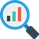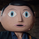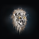# Quantitative Qualitative Estimation (QQE)

3092 views
3092
The indicator QQE, is an interesting tool based on a Relative Strength Index ( RSI ). While the original RSI is often used as a pointer for overbought or oversold market phases, the QQE provides additional information. Use the QQE to display trend direction and trend strength.

For me this is one of the most important indicator for Trend Following.

The histogram does not differ from the original QQE! The developer has adapted the scaling so that the central horizontal level is zero. It has no effect to the result, but is much more convenient to analyze the trend.

Main Signals

Background changes when the black line crosses the grey line.

Identify the trend direction

Singal turns green while the main QQE trendline is above the zero line and red while it is below.

This works best in the major timeframes like Daily or Weekly.
You can activate this signal in the settings.
NYSE:THO

Identify the trend strength

_Histogram Colors_
Green (above 10): bullish
Red (below -10): bearish
Yellow: flat

It is not a buy or sell signal when the color of the histogram changes. It only says that one side could gained the advantage.

If you use a large timeframe like Monthly, you can reduce the number of false signal by setting the SF (Slow Factor) from 5 (default) to 1.
S&P 500 , Monthly

Please always remember, there is no holy grail indicator!
...but this one defines trends quite accurately.
Comment removed.berlinguyinca
@berlinguyinca, Hi, no I don't giveaway the source code. Regards, Patrickberlinguyinca
@berlinguyinca, @fyntrade LOL nice copy ...

study("QQERSX")

RSI_Period = input(14,title='RSI Length')
SF = input(5,title='RSI Smoothing')
QQE=input(4.238,title='Fast QQE Factor')
ThreshHold=input(10,title="Thresh-hold")
//
sQQEx = input(false,title="Show Smooth RSI, QQE Signal crosses")
sQQEz = input(false,title="Show Smooth RSI Zero crosses")
sQQEc = input(false,title="Show Smooth RSI Thresh Hold Channel Exits")

src = input(close,title="RSI Source")
//

//
Wilders_Period = RSI_Period * 2 - 1

Rsi = rsi(src,RSI_Period)
RsiMa = ema(Rsi, SF)
AtrRsi = abs(RsiMa - RsiMa)
MaAtrRsi = ema(AtrRsi, Wilders_Period)
dar = ema(MaAtrRsi,Wilders_Period) * QQE

longband = 0.0
shortband=0.0
trend = 0

DeltaFastAtrRsi= dar
RSIndex=RsiMa
newshortband= RSIndex + DeltaFastAtrRsi
newlongband= RSIndex - DeltaFastAtrRsi
longband := RSIndex > longband and RSIndex > longband? max(longband,newlongband):newlongband
shortband := RSIndex < shortband and RSIndex < shortband? min(shortband, newshortband):newshortband
trend := cross(RSIndex, shortband)?1:cross(longband, RSIndex)?-1:nz(trend,1)
FastAtrRsiTL = trend==1? longband: shortband

//
// Find all the QQE Crosses
QQExlong = 0, QQExlong := nz(QQExlong)
QQExshort = 0, QQExshort := nz(QQExshort)
QQExlong := sQQEx and FastAtrRsiTL< RSIndex ? QQExlong+1 : 0
QQExshort := sQQEx and FastAtrRsiTL> RSIndex ? QQExshort+1 : 0
// Zero cross
QQEzlong = 0, QQEzlong := nz(QQEzlong)
QQEzshort = 0, QQEzshort := nz(QQEzshort)
QQEzlong := sQQEz and RSIndex>=50 ? QQEzlong+1 : 0
QQEzshort := sQQEz and RSIndex<50 ? QQEzshort+1 : 0
//
QQEclong = 0, QQEclong := nz(QQEclong)
QQEcshort = 0, QQEcshort := nz(QQEcshort)
QQEclong := sQQEc and RSIndex>(50+ThreshHold) ? QQEclong+1 : 0
QQEcshort := sQQEc and RSIndex<(50-ThreshHold) ? QQEcshort+1 : 0

// QQE exit from Thresh Hold Channel
plotshape(sQQEc and QQEclong==1 ? RsiMa-50:na , title="QQE XC Over Channel", style=shape.diamond, location=location.absolute, color=olive, transp=0, size=size.small, offset=0)
plotshape(sQQEc and QQEcshort==1 ? RsiMa-50:na , title="QQE XC Under Channel", style=shape.diamond, location=location.absolute, color=red, transp=0, size=size.small, offset=0)
// QQE crosses
plotshape(sQQEx and QQExlong==1 ? FastAtrRsiTL-50:na , title="QQE XQ Cross Over", style=shape.circle, location=location.absolute, color=lime, transp=0, size=size.small,offset=-1)
plotshape(sQQEx and QQExshort==1 ? FastAtrRsiTL-50 : na , title="QQE XQ Cross Under", style=shape.circle, location=location.absolute, color=blue, transp=0, size=size.small,offset=-1)
// Signal crosses zero line
plotshape(sQQEz and QQEzlong==1? RsiMa-50:na, title="QQE XZ Zero Cross Over", style=shape.square, location=location.absolute, color=aqua, transp=0, size=size.small,offset=0)
plotshape(sQQEz and QQEzshort==1? RsiMa-50:na, title="QQE XZ Zero Cross Under", style=shape.square, location=location.